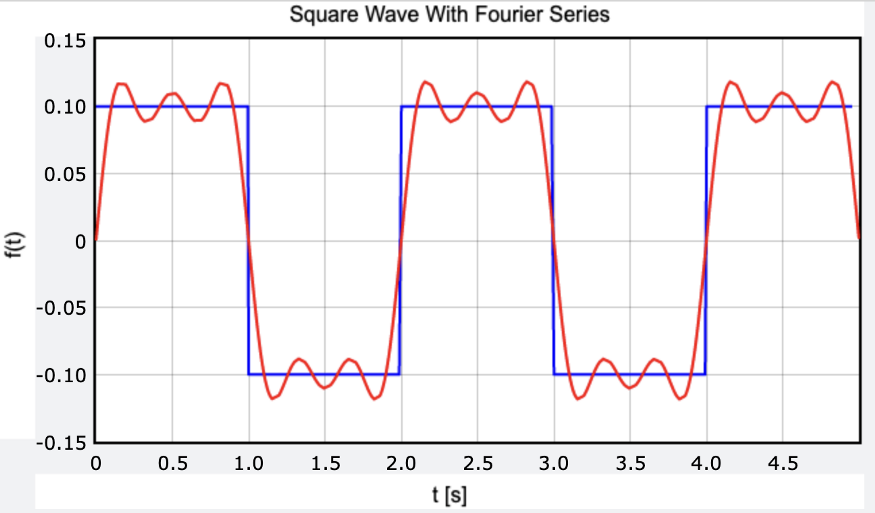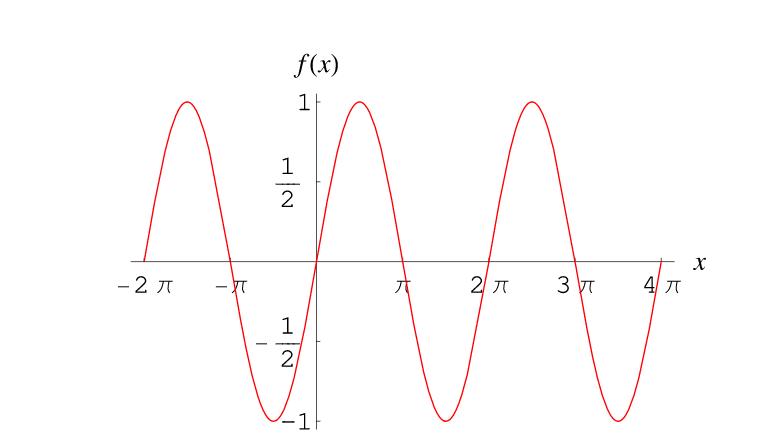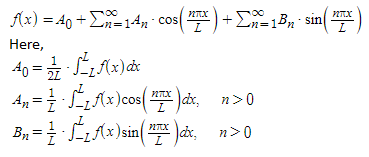# Fourier – Making Waves Study Guide

INTRODUCTION

In the domain of science and engineering, there are several phenomenons that are periodic in nature. For instance, sound waves, vibrations in structures, as well as current and voltage in AC circuits are examples. To study these things, it is essential to break them down into functions that can be analyzed. Fourier analysis is the process used to understand the constituent components of fundamental and harmonic frequencies in these systems.Source

## WHAT IS THE FOURIER SERIES?

A Fourier series is used to represent a periodic function f(x) as an infinite sum of sine and cosine functions. The study of the Fourier series is known as harmonic analysis. It is a highly useful tool to analyze arbitrary periodic functions by breaking them up into simpler terms that can be solved individually and then combined to solve the original problem.

## PERIODIC FUNCTIONS

A function is a unique relationship between members of two different sets. A periodic function is a relationship that repeats itself over a set of values. Consider the plot of the periodic function. F(x) = f(x np) where p is the period and n is a set of whole numbers n = 1, 2, … and so on. Here the graph shows a sine function with the least period of 2π as well as the periods -2π, 4π, 6π, etc.Source

Consider the functions sin(x) and sin(2x). The figure below shows each function as a waveform plotted on a graph. When you add the two, you make a new wave.Source

Complicated waves in many systems can be analyzed by breaking them down into smaller functions that can be solved individually and added up later. Consider the square wave shown below, for example:Source

It is possible to use sine waves to make a square wave. To that, we start with a sin(x) function:Source

Now take a new function sin(3x)/3 :Source

Add the two functions sin(x) sin(3x)/3 to generate this wave:Source

Now consider the wave of the function sin(5x)/5:Source

Add the above functions sin(x) sin(3x)/3 sin(5x)/5 to get:Source

Similarly if you add 20 more sine waves sin(x) sin(3x)/3 sin(5x)/5 … sin(39x)/39 we get a wave that’s close:

If this process continues infinitely, we will arrive at a square wave, which is the principle behind the Fourier series. The same process can be used to approximate a lot of different functions like the ones shown below:Source

## THE FOURIER SERIES FORMULA

The Fourier series formula for a function f(x) (such as the square wave in the above example) between the interval [-L, L] is given by:Source

## HARMONIC ANALYSIS

Fourier series is a branch of harmonic analysis. In music, if a note has a frequency of (f) which is called the fundamental frequency, its integer multiples such as 2f, 3f, 4f so on are called harmonics. These harmonics are where the instrument playing the note has its peak frequencies. For example, if the fundamental frequency of the first harmonic is 50 Hz, the second harmonic will be 100 Hz, and the third harmonic will be 150 Hz.Source

# CONCLUSION:

• Science and engineering problems often encounter phenomenons that repeat periodically.
• To analyze complex periodic functions, it is essential to break them down into smaller functions.
• A Fourier series is used to represent a periodic function f(x) as an infinite sum of sine and cosine functions.
• Fourier series is a branch of harmonic analysis.

We hope you enjoyed studying this lesson and learned something cool about The Fourier Series! Join our Discord community to get any questions you may have answered and to engage with other students just like you! We promise it makes studying much more fun! 😎

## SOURCES:

1. Fourier Series:
2. https://mathworld.wolfram.com/FourierSeries.html#:~:text=A%20Fourier%20series%20is%20an,the%20sine%20and%20cosine%20functions. Accessed 25 Feb 2022.
3. Harmonic Analysis: https://mathworld.wolfram.com/HarmonicAnalysis.html. Accessed 25 Feb 2022.
4. Periodic Function: https://mathworld.wolfram.com/PeriodicFunction.html. Accessed 25 Feb 2022.
5. Fourier Series: https://byjus.com/maths/fourier-series/. Accessed 25 Feb 2022.
6. Fourier Series: https://www.mathsisfun.com/calculus/fourier-series.html. Accessed 25 Feb 2022.
7. Fundamental and Harmonic Frequencies: https://www.teachmeaudio.com/recording/sound-reproduction/fundamental-harmonic-frequencies. Accessed 25 Feb 2022.
8. Sound Spectrum: https://newt.phys.unsw.edu.au/jw/sound.spectrum.html Accessed 25 Feb 2022.
]]>